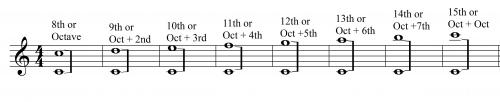Intervals

A Interval is the distance between two notes. In building the major scale we discussed two intervals: the half step and whole step. There are intervals that go beyond the half step and whole step and we will discuss each of those intervals in this lesson.

Diatonic Intervals

There are two types of intervals: Diatonic and Chromatic/Real Intervals. First, we will discuss diatonic intervals.

Figure: Melodic and Harmonic Diatonic IntervalsThe easiest method for determining intervals is of a scale(diatonic intervals) is labeling each note with a letter or a number.

Figure: C major scale with caratsThe following chart will help determine the diatonic interval.

Compound Intervals

Compound intervals are intervals beyond the octave. It is relatively easy to find the compound interval. All intervals are more than an octave so we add the scale degree + the octave.

Figure: Compound IntervalsInterval Inverses

When an interval is flipped an octave that interval takes on a different name because of the new distance.

A unison flipped an octave is an octave.

A 2nd flipped an octave is a 7th

A 3rd flipped an octave is a 6th

A 4th flipped an octave is a 5th

Video Lesson #1 – Diatonic Intervals

Video Lesson #2 – Interval Inversion